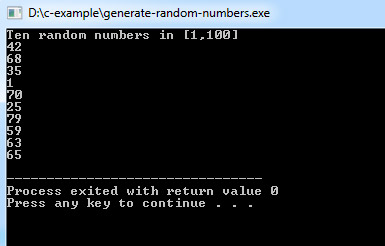# C Program to Generate Random Numbers

This C program generates numbers randomly using random function.

In this program for loop is used to call rand() function multiple times.

Program:
``````#include <stdio.h>
#include <stdlib.h>

int main()
{
int c, n;
printf("Ten random numbers in [1,100]\n");

for (c = 1; c <= 10; c++)
{
n = rand()%100 + 1;
printf("%d\n", n);
}

return 0;
}``````
Program Output: